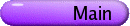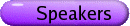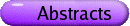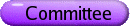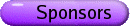Algebraic Combinatorics Org: Nantel Bergeron (York), Christophe Hohlweg (Fields Institute) and Michael Zabrocki (York)[PDF] MARCELO AGUIAR, Texas A&M University Hopf monoids in Species and associated Hopf algebras [PDF] The recent literature has seen the emergence and profusion of certain Hopf algebras of a combinatorial nature. We propose a general framework for the construction and study of these Hopf algebras. We study the tensor category of species and relate it to the tensor category of graded vector spaces by means of bilax tensor functors. Constructions of graded Hopf algebras from Hopf monoids in species are derived. We use the geometry and combinatorics of the Coxeter complex of type A to construct Hopf monoids. The corresponding Hopf algebras include those of symmetric functions, quasisymmetric functions, noncommutative symmetric functions, other Hopf algebras of prominence in the recent literature, and new ones. The categorical approach yields uniform deformations and higher-dimensional generalizations of all these objects. FRANÇOIS BERGERON, UQAM, Dépt. Math, C.P. 8888, Succ. Centre-Ville, Montréal, H3C 3P8 Harmonics for Steenrod Operators [PDF] We present joint work with A. Garsia and N. Wallach concerning the space of harmonic polynomials for deformations of Steenrod Operators. On the side this gives rise to the study of a new variety of skewed versions of coinvariant spaces for reflection groups. PHILIPPE CHOQUETTE, York University, 4700 Keele St., Toronto, Ontario, M3J 1P3 The ring of r-quasisymmetric polynomials [PDF] We present some results about the ring of r-quasisymmetric polynomials. We discuss the quotients of these rings and morphism between them. IAN GOULDEN, University of Waterloo, Waterloo Combinatorics and intersection number conjectures [PDF] The purpose of this talk is to explain the combinatorial portion of a long-standing collaboration with David Jackson and Ravi Vakil. This is concerned with intersection numbers for moduli spaces and a combinatorialization by Vakil, an algebraic geometer. In particular, we describe Kazarian and Lando's recent proof of Witten's Conjecture, and our recent proof of the lg-Conjecture, as well as our recent proof of various infinite cases of Faber's conjecture. Our combinatorial methods feature generating series for various classes of labelled rooted trees. AARON LAUVE, UQAM, LaCIM, C.P. 8888, Succ. Centre-Ville, Montréal, H3C 3P8 Noncommutative invariants and coinvariants of the symmetric group [PDF] The algebras NCSymn and Symn (n Î N+) are defined to be the Sn-invariants inside Q áAn ñ (resp. Q[Xn]), the polynomial functions on a noncommutative alphabet An (resp. commutative, Xn) of cardinality n. The abelianization (ai® xi) realizes Symn as a quotient of NCSymn. Here, we view it as a subspace. Some surprising identities on the ordinary generating function for the Bell numbers appear as an immediate corollary. In case n=¥, we obtain new information on the (Hopf) algebraic structure of NCSymn. Time permitting, we outline similar results for Hivert's r-QSymn algebras (r,n Î N+ È{¥}) and their noncommutative analogues. Joint work with F. Bergeron. ROSA ORELLANA, Dartmouth Hopf algebra of uniform block permutations [PDF] In this talk I will present the Hopf algebra of uniform block permutations and show that it is self-dual, free, and cofree. These results are closely related to the fact that uniform block permutations form a factorizable inverse monoid. This Hopf algebra contains the Hopf algebra of permutations of Malvenuto and Reutenauer and the Hopf algebra of symmetric functions in non-commuting variables of Gebhard, Rosas, and Sagan. MUGE TASKIN, York University, 2025 TEL Building, North York, ON, M3J 1P3 The properties of four partial orders on standard Young tableaux [PDF] Standard Young tableaux have been well knowm with their connection with the representation theory of symmetric group and special linear algebra sln. In this talk we will focus on the following four partial orders which are induced from this connection: weak, KL, geometric and chain orders. After recalling their definitions and some of their crucial properties, we will discuss three main results about these orders. The first one is related to the product in a Hopf algebra of tableaux defined by Poirier and Reutenauer. The second one is about the homotopy type of their proper parts. The last one addresses two of these orders which can be defined on the skew tableaux having fixed inner boundary, and similarly analyzes their homotopy type and Möbius function. The talk will further include some preliminary results about domino tableaux, which are also related to the representation theory of symplectic algebra sp2n and orthogonal algebra so2n+1. HUGH THOMAS, Department of Mathematics and Statistics, University of New Brunswick, Fredericton, NB A combinatorial rule for (co)minuscule Schubert calculus [PDF] I will discuss a root system uniform, concise combinatorial rule for Schubert calculus in minuscule and cominuscule flag manifolds G/P. (The latter are also known as compact Hermitian symmetric spaces.) We connect this geometry to work of Proctor in poset combinatorics, thereby generalizing Schutzenberger's jeu de taquin formulation of the Littlewood-Richardson rule for computing intersection numbers of Grassmannian Schubert varieties. I will explain the rule, give some background, and, time permitting, give some idea of the proof, including the notion, which we introduce, of cominuscule recursion, which is a general technique which relating the structure constants for different Lie types. This talk presents joint work with Alex Yong, and is based on the preprint (with the same title) math.AG/0608276. STEPHANIE VAN WILLIGENBURG, University of British Columbia A combinatorial classification of skew Schur functions [PDF] We present a single operation for constructing skew diagrams whose corresponding skew Schur functions are equal. This combinatorial operation naturally generalises and unifies all results of this type to date. Moreover, our operation suggests a closely-related condition that we conjecture is necessary and sufficient for skew diagrams to yield equal skew Schur functions.